# COMPUTER PROGRAMMING Lab7 Exercise 1 Decimal to hex

• Slides: 10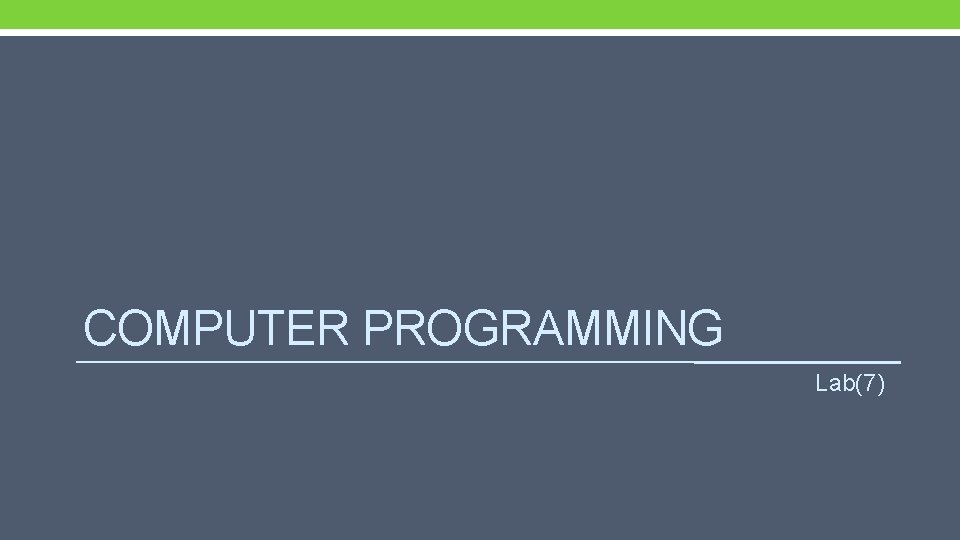COMPUTER PROGRAMMING Lab(7)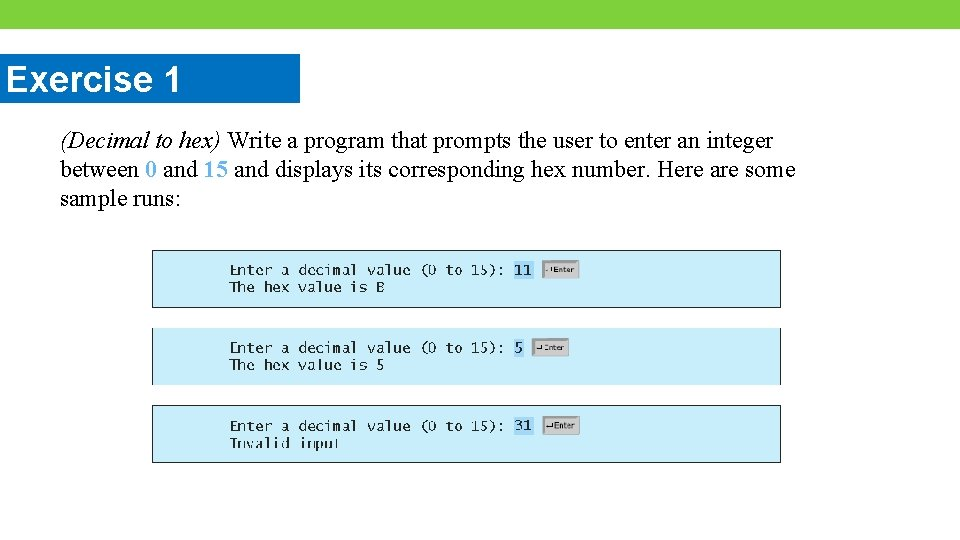Exercise 1 (Decimal to hex) Write a program that prompts the user to enter an integer between 0 and 15 and displays its corresponding hex number. Here are some sample runs: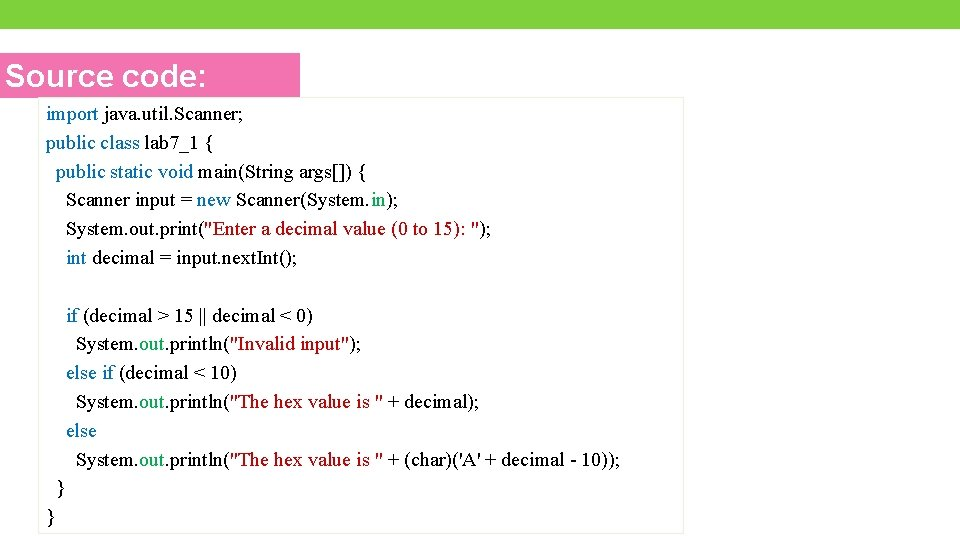Source code: import java. util. Scanner; public class lab 7_1 { public static void main(String args[]) { Scanner input = new Scanner(System. in); System. out. print("Enter a decimal value (0 to 15): "); int decimal = input. next. Int(); if (decimal > 15 || decimal < 0) System. out. println("Invalid input"); else if (decimal < 10) System. out. println("The hex value is " + decimal); else System. out. println("The hex value is " + (char)('A' + decimal - 10)); } }Output:Exercise 2 (Financial: compare costs) Suppose you shop for rice in two different packages. You would like to write a program to compare the cost. The program prompts the user to enter the weight and price of the each package and displays the one with the better price. Here is a sample run: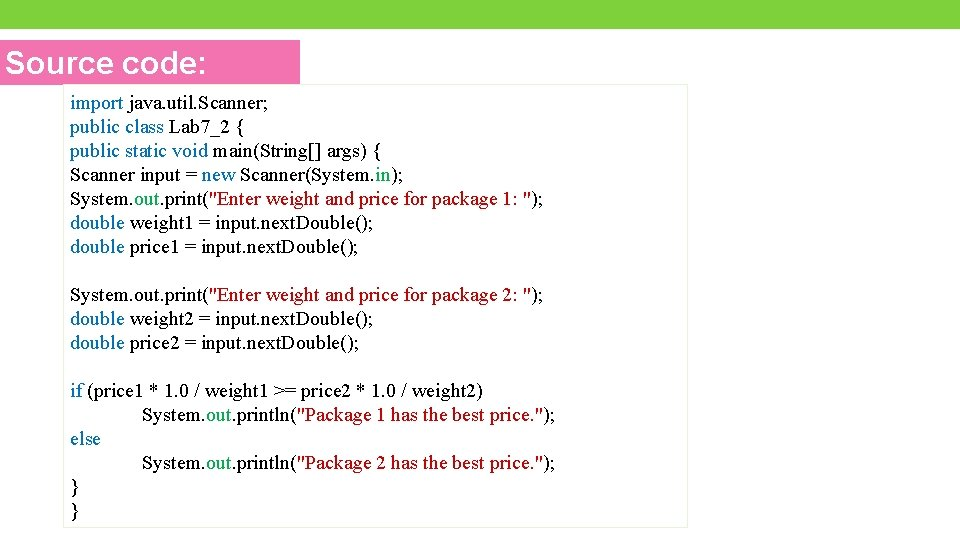Source code: import java. util. Scanner; public class Lab 7_2 { public static void main(String[] args) { Scanner input = new Scanner(System. in); System. out. print("Enter weight and price for package 1: "); double weight 1 = input. next. Double(); double price 1 = input. next. Double(); System. out. print("Enter weight and price for package 2: "); double weight 2 = input. next. Double(); double price 2 = input. next. Double(); if (price 1 * 1. 0 / weight 1 >= price 2 * 1. 0 / weight 2) System. out. println("Package 1 has the best price. "); else System. out. println("Package 2 has the best price. "); } }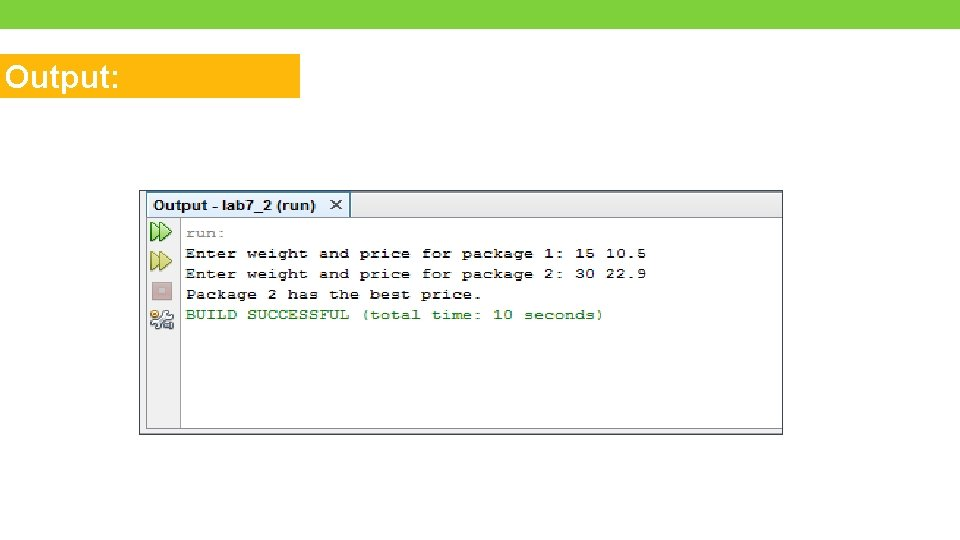Output:Exercise 3 (Find the Largest Number) The process of finding the largest value is used frequently in computer applications. Write a program that reads 3 integers from the console and print the largest integer between them. Here is a sample run:Source code: Scanner input = new Scanner(System. in); System. out. print("Enter the first integer: "); int x = input. next. Int(); System. out. print("Enter the second integer: "); int y = input. next. Int(); System. out. print("Enter the third integer: "); int z = input. next. Int(); if ( x > y && x > z ) System. out. println("The largest number between ["+x+", "+y+", "+z+"] is "+ x); else if ( y > x && y > z ) System. out. println("The largest number between ["+x+", "+y+", "+z+"] is "+ y); else if ( z > x && z > y ) System. out. println("The largest number between ["+x+", "+y+", "+z+"] is "+ z); else System. out. println("Entered numbers are not distinct");Output: